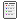###Author Topic: BI Systems Must Integrate and Consolidate Any Data  (Read 543 times)

#### Shakib

• Newbie
•• Posts: 24##### BI Systems Must Integrate and Consolidate Any Data
« on: September 28, 2018, 04:37:28 PM »
If data only could be stored as numbers in spreadsheet cells, then consolidation would pose a significant problem for an Excel BI. But in fact, spreadsheet cells that rely on an Excel BI don't contain data, they contain formulas linked to data.

And the data can come from any available source, and from any number of  sources.

In an Excel BI, data typically is stored in a multidimensional (OLAP) database, which is very similar to a group of multidimensional Excel workbooks. Each of these workbook-like containers is called a cube. Most cubes contain formulas that consolidate the data automatically.

Cube formulas can support more sophisticated calculations. For example, they can calculate ratios, do currency translation, discount cash flows, calculate logarithms, and so on...just like a workbook can.

But they can do it in multiple dimensions. Automatically.

Excel users enter spreadsheet formulas that return numbers, text, or calculated values from any cell in any of those cubes. For example, a formula like this...

=GETDATA("Sales", "Units", "Hats", "Store 19", "Oct-2007")

...returns unit sales information consolidated by product line and region for the month shown. And this formula...

=GETDATA("Sales", "Sales USD", "Hats", "Store 19", "Oct-2007 YTD")

...returns year-to-date sales in US dollars for the same product line and region.

As with any Excel formula, of course, OLAP formulas typically reference cells that contain labels like "Units", "Hats", and other arguments. The formulas typically don't contain the arguments themselves. Using this approach, you could replace "Hats" with "Coats" in a cell, and then recalculate, to create a report about coats.

To my knowledge, no Excel BI product has a function named GETDATA. But they all have functions that work about like the GETDATA function shown here.

Collection from